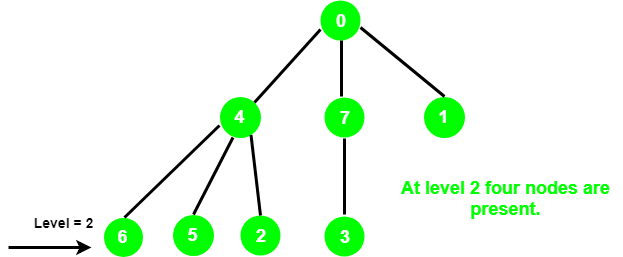# Count the number of nodes at a given level in a tree using DFS

Given an integer l and a tree represented as an undirected graph rooted at vertex 0. The task is to print the number of nodes present at level l.

Examples:

Input: l = 2Output: 4

## Recommended: Please try your approach on {IDE} first, before moving on to the solution.

We have already discussed the BFS approach, in this post we will solve it using DFS.

Approach: The idea is to traverse the graph in a DFS manner. Take two variables, count and curr_level. Whenever the curr_level = l increment the value of the count.

Below is the implementation of the above approach:

 `// C++ implementation of the approach ` `#include ` `using` `namespace` `std; ` ` `  `// Class to represent a graph ` `class` `Graph { ` ` `  `    ``// No. of vertices ` `    ``int` `V; ` ` `  `    ``// Pointer to an array containing ` `    ``// adjacency lists ` `    ``list<``int``>* adj; ` ` `  `    ``// A function used by NumOfNodes ` `    ``void` `DFS(vector<``bool``>& visited, ``int` `src, ``int``& curr_level, ` `             ``int` `level, ``int``& NumberOfNodes); ` ` `  `public``: ` `    ``// Constructor ` `    ``Graph(``int` `V); ` ` `  `    ``// Function to add an edge to graph ` `    ``void` `addEdge(``int` `src, ``int` `des); ` ` `  `    ``// Returns the no. of nodes ` `    ``int` `NumOfNodes(``int` `level); ` `}; ` ` `  `Graph::Graph(``int` `V) ` `{ ` `    ``this``->V = V; ` `    ``adj = ``new` `list<``int``>[V]; ` `} ` ` `  `void` `Graph::addEdge(``int` `src, ``int` `des) ` `{ ` `    ``adj[src].push_back(des); ` `    ``adj[des].push_back(src); ` `} ` ` `  `// DFS function to keep track of ` `// number of nodes ` `void` `Graph::DFS(vector<``bool``>& visited, ``int` `src, ``int``& curr_level, ` `                ``int` `level, ``int``& NumberOfNodes) ` `{ ` `    ``// Mark the current vertex as visited ` `    ``visited[src] = ``true``; ` ` `  `    ``// If current level is equal ` `    ``// to the given level, increment ` `    ``// the no. of nodes ` `    ``if` `(level == curr_level) { ` `        ``NumberOfNodes++; ` `    ``} ` `    ``else` `if` `(level < curr_level) ` `        ``return``; ` `    ``else` `{ ` `        ``list<``int``>::iterator i; ` ` `  `        ``// Recur for the vertices ` `        ``// adjacent to the current vertex ` `        ``for` `(i = adj[src].begin(); i != adj[src].end(); i++) { ` `            ``if` `(!visited[*i]) { ` `                ``curr_level++; ` `                ``DFS(visited, *i, curr_level, level, NumberOfNodes); ` `            ``} ` `        ``} ` `    ``} ` `    ``curr_level--; ` `} ` ` `  `// Function to return the number of nodes ` `int` `Graph::NumOfNodes(``int` `level) ` `{ ` `    ``// To keep track of current level ` `    ``int` `curr_level = 0; ` ` `  `    ``// For keeping track of visited ` `    ``// nodes in DFS ` `    ``vector<``bool``> visited(V, ``false``); ` ` `  `    ``// To store count of nodes at a ` `    ``// given level ` `    ``int` `NumberOfNodes = 0; ` ` `  `    ``DFS(visited, 0, curr_level, level, NumberOfNodes); ` ` `  `    ``return` `NumberOfNodes; ` `} ` ` `  `// Driver code ` `int` `main() ` `{ ` `    ``int` `V = 8; ` ` `  `    ``Graph g(8); ` `    ``g.addEdge(0, 1); ` `    ``g.addEdge(0, 4); ` `    ``g.addEdge(0, 7); ` `    ``g.addEdge(4, 6); ` `    ``g.addEdge(4, 5); ` `    ``g.addEdge(4, 2); ` `    ``g.addEdge(7, 3); ` ` `  `    ``int` `level = 2; ` ` `  `    ``cout << g.NumOfNodes(level); ` ` `  `    ``return` `0; ` `} `

Output:

```4
```

Attention reader! Don’t stop learning now. Get hold of all the important DSA concepts with the DSA Self Paced Course at a student-friendly price and become industry ready.

My Personal Notes arrow_drop_upCheck out this Author's contributed articles.

If you like GeeksforGeeks and would like to contribute, you can also write an article using contribute.geeksforgeeks.org or mail your article to contribute@geeksforgeeks.org. See your article appearing on the GeeksforGeeks main page and help other Geeks.

Please Improve this article if you find anything incorrect by clicking on the "Improve Article" button below.

Article Tags :
Practice Tags :

Be the First to upvote.

Please write to us at contribute@geeksforgeeks.org to report any issue with the above content.